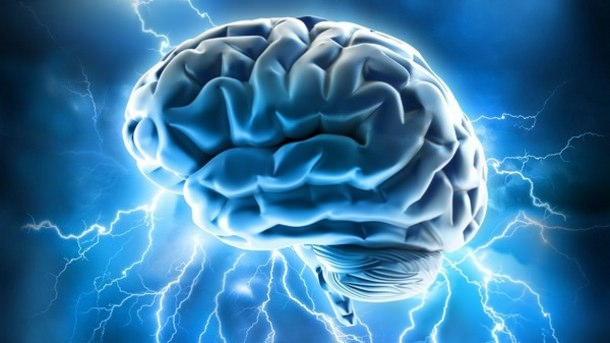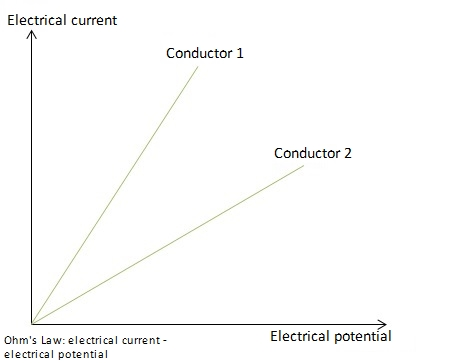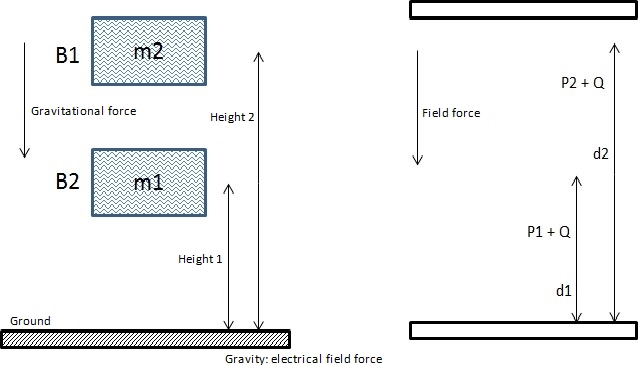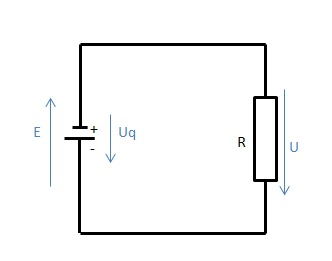The following article explains the different aspects of electrical engineering, their relation to the human body, and the relationship between different variables.Image: “brain power” by Allan Ajifo. License: CC BY 2.0

## Electric Charge and Electric Current

A flow field refers to the directed flow of particles in a medium. Under a constant particle flow, the number of particles that enter the flow field is equal to the number of particles leaving it and is referred to as a stationary flow field. Stationary electric field is characterized by particles carrying electric charges. Electric charge is generated when matter experiences force under an electromagnetic field.

Such fields are formed, for example, in metals or aqueous solutions found in the human body, which consists of approx. 60% water. The ions, which are electrically charged atoms or molecules, are responsible for the flow field.
All electrical charges are multiples of elementary charge that is no longer divisible. Since antiquity, it has been known that two different electric charges exist: positive and negative. The electron carries a single negative elementary charge:

QE = e = -1,602 × 10-19 C

I [A] ⇒ current in amperes
ΔQ [A*s], [C] ⇒ quantity of electricity (charge)
Δt [s] ⇒ time period
QE [C] ⇒ elementary charge

The unit of electric charge is the Coulomb [C]. Ions can carry multiple elementary charges and be positively or negatively charged. The laws of attraction between positive and negative ions apply to charges as well, i.e. like charges repel whereas unlike charges attract. Unit of a quantity of electricity is defined as the charge of one coulomb, which is 6. 242 × 1018 times the charge of a single electron.

Electric charge induces reactions of variable intensity and is referred to as the physical quantity of electric current. The flow of electric charge is electric current. Ampere is the unit used to measure electric current using the instrument known as an ammeter. The ammeter is connected in series with the battery. One ampere is the constant current that produces an attractive force equal to 0.2 N × 10-6 per meter of length between two straight, parallel conductors of infinite length and negligible circular cross-sections placed one meter apart in a vacuum.

The electric current is defined as the ratio between the quantity of electricity (charge) and the duration of its flow:

I = ΔQ / Δt

Based on the above equation, the electric current is directly proportional to the amount of charge. Electric currents of about 10 mA have lethal consequences for humans.

Note: The electric current corresponds to the periodic change in the electric charge. If the timing of the charge generated in the cross-sectional area is known, we can calculate the associated current simply by differentiating the charge function.

## Electric Potential

U [V] ⇒ electric potential (voltage)
R [Ω] ⇒ electric resistance
G [S], [1 / Ω] ⇒ electric conductance

The electrical potential is always measured between two points. In an electric circuit, the current only flows when there is a voltage between its poles. The electric potential is measured using a voltmeter. The voltmeter is connected in parallel with the component, whose potential is measured.

The voltage (V or U) between the two points of a conductor is defined by the ratio of the power generated to the current flowing through the conductor. The electric potential is expressed in SI units:

1 Volt = 1 Watt / 1 Ampere

## Principles of Electric Circuit

### Ohm’s law

In 1827, the physicist Georg Simon Ohm investigated the dependence of electric charge on the current in a conductor piece. He found that the electric current-voltage U passing through a conductor between any two points is directly proportional to the potential difference across the two points. He described Ohm’s Law as follows:

U ∼ I R = U / I

Ohm’s Law is only valid when the temperature remains constant.The quotient of voltage and current is called electrical resistance. It is specified by the unit Ohm [Ω]. The electrical resistance of a metallic conductor is constant only at a steady temperature. When the temperature changes, resistance changes as well. With increasing temperature, the voltage and current also rise, and thus it is the resistance of the conductor under constant temperature and the electric potential between two points in the conductor. Thus a higher level of current flows through a battery with higher voltage, and vice versa. However, it varies, such that the resistance of solid conductors increases with increasing temperature. The resistance of liquid conductors decreases with increasing temperature. Ohm’s law cannot be applied for electricity in gases.

Resistance varies from one conductor to another, as shown in the graph above: conductor 2 has a higher resistance than conductor 1. These two conductors obey Ohm’s law, according to which the electric current increases with increasing potential. Such conductors are referred as Ohmic conductors, and those that do not obey Ohm’s law are called non-Ohmic conductors.

Electrical conductivity (conductance) is the reciprocal of resistance. It is specified by the unit Siemens.
Example: The current in the body depends on the voltage between the contact points and the body’s resistance. The body’s resistance decreases with increasing voltage and depends on the routes of the current flow. The resistance of skin amounts to several thousand Ohms, but at high voltages, it may drop to zero. The current path between the left and right hands carries a resistance of approximately 650 Ohms.

### Coulomb’s law

Coulomb’s Law is used to calculate the electric forces.

E [V / m] ⇒ electric field
F ⇒ resulting force on different charges
ε [F / m] ⇒ permittivity

The most important equations which describe the electric potential force between two charged objects in electrostatic fields are listed below:

Force on quantity charge Q in the area of an intensity field:

F = Q × E

Result of the force of electrical charge of the bodies Q1 and Q2, which are separated by distance ‘a’ as defined by the equation governing Coulomb’s Law:

F = Q2 / (2 × ε × A) = (D × E × A) / 2

Two oppositely charged particles generate attractive potential. However, a repulsive potential is generated if both particles are of the same charge—for example, both are positive or both are negative. It is similar to Newton’s Law of Gravitation, in which, however, only attractive forces are possible.

Force between plane-parallel plates, such as plate capacitor:

## Potential

Since water and water-flow behave similar to an electrical current, the movement of charge carriers can, therefore, be explained in further detail.As shown in the illustration, two containers are filled with water containers B1 and B2. The orifices in both containers are located at heights h1 and h2 above the ground. Gravity acts towards the earth. The potential energy of mass situated above ground is calculated by the gravitational force ‘g’:

W = m × g × h.

It can be used, for example, when the valves are opened and the water drains out.

A reference point for each potential specification (in this case the height) is needed. W represents the work that is performed in order to adjust the mass to the corresponding height. Therefore, the gravity or gravitational potential of a point with respect to another point corresponds to the work performed against gravity to bring any mass from the reference point to the receiving point, divided by the quantity of the mass.

In a flow field, a positive charge of the unit Q is located at each point P1 and P2. The current flow and the flow of the two charges are under the electric field force. Here, it has been randomly drawn as acting downwards from above, in order to facilitate a direct comparison with the gravitational field.

It could also act upwards, when the charges ‘fall upwards’ as they are only subject to the field force and, due to their very low mass, are not affected by the gravitational force. Parameters defining the gravitational potential apply equally to the electric flow, which is, therefore, known as electric potential wherein the masses are replaced by the charges.

Note: The electric potential energy of a reference point, in relation to another (the receiving point), corresponds to the work performed against the electric field strength to carry the charge from the reference point to the receiving point, divided by the quantity of this charge.

## Electric Circuit

All electrical elements in electric flow fields or electrical circuits, in which the flowing charge carriers absorb energy, are known as current or voltage sources. All the components, in which the flowing charge carriers release energy are referred to as the electric circuit load. The electromotive force is generated when a voltage or a potential drop occurs on the electric load.

In the last illustration, all charge carriers move under the influence of the field force in the direction of the lower border of the flow field, releasing electrical energy. This energy is exhausted upon reaching the lower boundary electrodes.

Energy release from the flow field again requires an increase in the charge carriers to a higher energy potential once again. Therefore, the charge carriers need to be raised to the upper boundary electrode, in order for them to pass down the electric field, to release electrical energy. Continuous repetition of this leads to a continuous release of electrical energy to the flow field.

However, the precise method required to relocate the charge carriers either back or upwards is unclear. As this cannot be done in the midst of the flow field, external intervention is needed. For example, the charge carriers can be intercepted after arriving at the lower boundary electrode and traveling via an electric access line to a power source where the necessary energy is supplied by the electromotive force and then transported via a second connecting line to the upper border of the flow field.

Continuous circular flow is thus maintained in a system, which is called the electrical circuit. It consists of two locally separate elements:

• The voltage source, in which the current-forming charge carriers receive energy.
• The electrical load, in which they release it again.The illustration shows the electrical circuit with the two core elements that are interconnected by electric leads. The circuit symbol that is used for the source here represents a battery. The electric load is represented by a resistor R, at which a potential drop occurs.

## Laws of Kirchhoff

Two laws of Kirchhoff play a significant role throughout the entire field of electrical engineering. Circuits are usually not as simply structured as shown previously but carry special junction points or current nodes.

### Kirchhoff’s current law or nodal rule

In a node, the sum of all currents is zero, i.e. at any node in an electrical circuit, the total current inflow is equal to the total current outflow at that node. The electric current is conserved and a stationary flow is generated at a node.

### Kirchhoff’s voltage law or loop rule

The sum of all voltages in any loop is equivalent to the sum of the potential drops in that loop, implying that whatever energy a charge Q starts within an electrical circuit is lost by the time the charge reaches the end. As each point on the circuit is characterized by a unique potential value, any number of loops should carry the same potential.

Example 1: We are familiar with the general physical principles governing the blood flow through the vascular system. Considering the heart as a node, the volume of blood that flows into the heart is equal to the amount that flows out again. Since blood is not a ‘Newtonian fluid’, based on the resistor, Kirchoff’s rules apply as follows:

Kirchoff’s first law, in connection with the vascular system of a human being, states that the flow resistance of all ‘consecutively connected’ blood vessels accumulates. Therefore, the overall resistance increases with the number of consecutive blood vessels.

Kirchoff’s second law states that the reciprocal value of the total resistance of parallel blood vessels is equal to the reciprocals of the individual resistors. The higher the number of parallel blood vessels, the greater is the decrease in total resistance.

Example 2: In pulmonary embolism, a pulmonary artery or arteriole, and therefore, one of the several parallel blood vessels is blocked and can no longer contribute to the reduction in the total flow resistance. Therefore, the total resistance of the pulmonary arteries increases (2nd Kirchhoff’s law).

In the second section on Electricity II, alternating current (AC) is discussed.

Learn. Apply. Retain.
Your path to achieve medical excellence.
Study for medical school and boards with Lecturio.